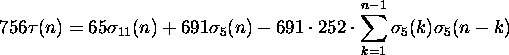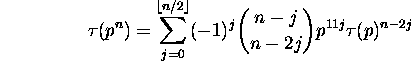### Computing Ramanujan's tau function

Our program is simple-minded and uses the formulawhen n is prime and the formulaHere σk(n) is the sum of the k-th powers of the divisors of n.
(See T.M. Apostol, Modular functions and Dirichlet series in number theory, 20-22, 140 and the MathWorld account.)

Enter n (0 < n ≤ 20000):